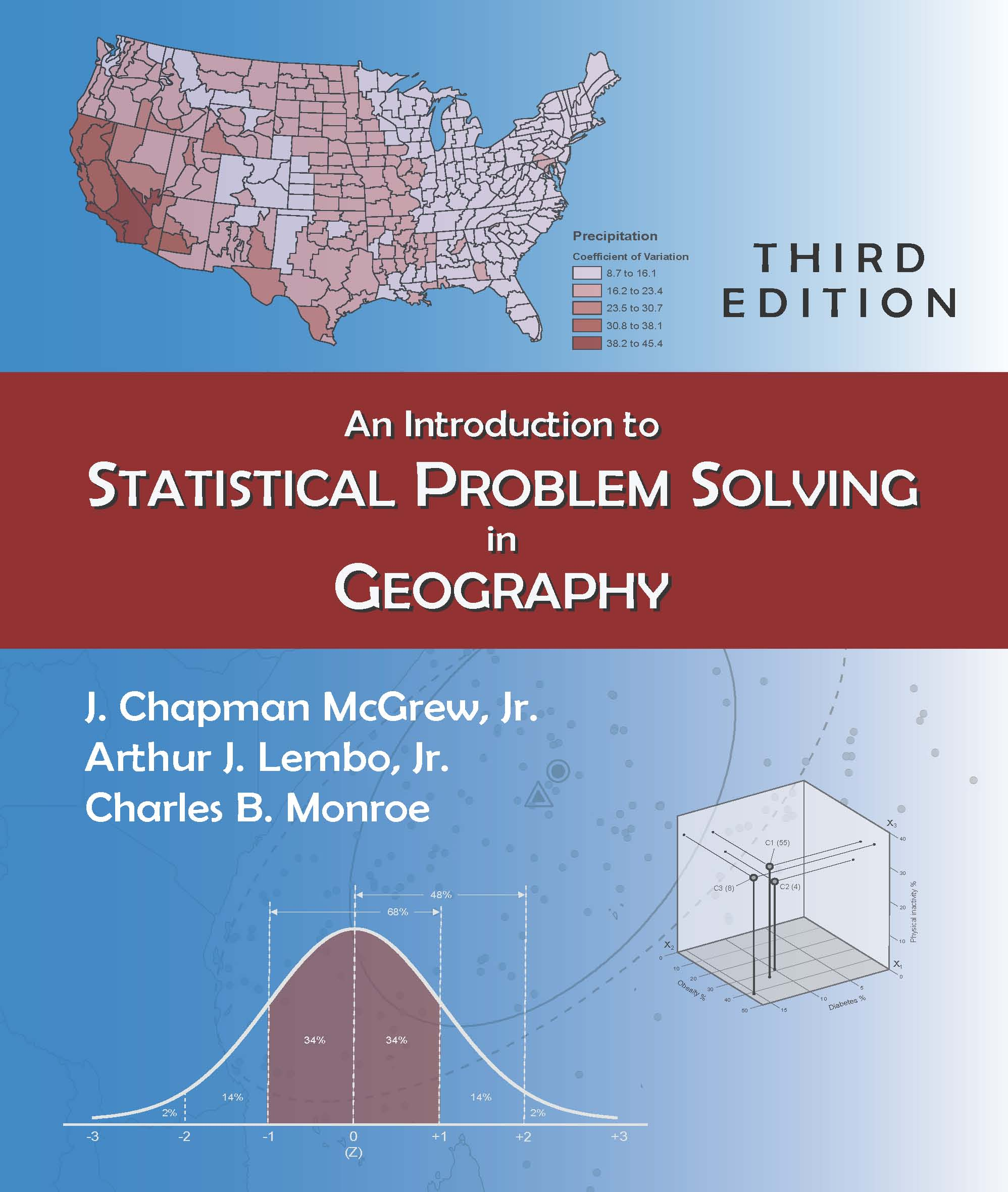320 pages, \$55.95 list
1-4786-1119-7
978-1-4786-1119-6
Instructor's resource materials available here
To obtain a username and password to access these materials, contact comps@waveland.com.
Student resource materials available here
eBook availability
New edition coming soon!
An Introduction to Statistical Problem Solving in Geography
Third Edition
Now in its third edition, this highly regarded text has firmly established itself as the definitive introduction to geographical statistics. Assuming no reader background in statistics, the authors lay out the proper role of statistical analysis and methods in human and physical geography. They delve into the calculation of descriptive summaries and graphics, the use of inferential statistics as exploratory and descriptive tools, ANOVA and Kruskal-Wallis tests and different spatial statistics to explore geographic patterns, inferential spatial statistics, and spatial autocorrelation and variograms.

The authors maintain an exploratory and investigative approach throughout, providing readers with real-world geographic issues and more than 50 map examples. Concepts are explained clearly and narratively without oversimplification. Each chapter concludes with a list of major goals and objectives. An epilogue offers over 150 geographic situations, inviting students to apply their new statistical skills to solve problems currently affecting our world. Data sets for many of the book's examples, appropriate for use with a variety of statistical and GIS software can be downloaded here.
Reactions
“I have thoroughly studied and tried out a number of textbooks for the third-year course, Statistical Methods for Geographers, and was disappointed. In many cases I found the explanations to be weak, often irrelevant, and confusing. The level of difficulty of other books, which were better written, was too high. This text seems to be exactly what I need. It strikes the right balance between rigor and accessibility. It contains some of the best explanations I could find for my students on key topics in statistics. The interesting practical applications supporting the core material and the numerous exercises at the end of the book are invaluable. It is the best textbook I could find on this subject at this level.” — Cristian Suteanu, Saint Mary’s University

“Rather than dreading statistics, this book shows students the value of a multitude of techniques and how to select the best one for a particular problem. Equally important, the authors show students how to interpret the results of their analysis.” — The Pennsylvania Geographer

“Social science statistics oftentimes is not focused on geography as a unique discipline with its own set of problems and applications. This text provides both the theory and application of statistics for undergraduate students in geography.” — Christopher Atkinson, University of North Dakota

“The book is great! A welcome resource for students who are just beginning geostatistics. The examples are contextually simple to understand without sacrificing too much theory.” — Peter Oduor, North Dakota State University

“This easy-to-understand book is a great resource for students. It is full of practical examples in plain English. The authors have presented and explained otherwise complicated topics of statistics in a manner that any college student can easily comprehend.” — Bhuiyan Alam, University of Toledo

"Without the McGrew text my course wouldn't be a 'geographic statistics' course. I love the book and my students do too." — Paul Sutton, University of Denver
Part I: BASIC STATISTICAL CONCEPTS IN GEOGRAPHY

1. Introduction: The Context of Statistical Techniques
The Role of Statistics in Geography / Examples of Statistical Problem Solving in Geography

2. Geographic Data: Characteristics and Preparation
Selected Dimensions of Geographic Data / Levels of Measurement / Measurement Concepts / Basic Classification Methods

Part II: DESCRIPTIVE PROBLEM SOLVING IN GEOGRAPHY

3. Descriptive Statistics and Graphics
Measures of Central Tendency / Measures of Dispersion and Variability / Measures of Shape or Relative Position / Selected Issues: Spatial Data and Descriptive Statistics

4. Descriptive Spatial Statistics
Spatial Measures of Central Tendency / Spatial Measures of Dispersio

Part III: THE TRANSITION TO INFERENTIAL PROBLEM SOLVING

5. Basics of Probability and Discrete Probability Distributions
Basic Probability Processes, Terms, and Concepts / The Binomial Distribution / The Geometric Distribution / The Poisson Distribution

6. Continuous Probability Distributions and Probability Mapping
The Normal Distribution / Probability Mapping

7. Basic Elements of Sampling
Sampling Concepts / Types of Probability Sampling / Spatial Sampling

8. Estimation in Sampling
Basic Concepts in Estimation / Confidence Intervals and Estimation / Geographic Examples of Confidence Interval Estimation / Sample Size Selection

Part IV: INFERENTIAL PROBLEM SOLVING IN GEOGRAPHY

9. Elements of Inferential Statistics
Terms and Concepts in Hypothesis Testing: One-Sample Difference of Means Test / One-Sample Difference of Proportions Test / Selected Issues in Inferential Testing

10. Two-Sample and Dependent-Sample (Matched-Pairs) Difference Tests
Two-Sample Difference of Means Test / Two-Sample Difference of Proportions Test / Dependent-Sample (Matched-Pairs) Difference Tests

11. Three-or-More-Sample Difference Tests: Analysis of Variance Methods
Analysis of Variance (ANOVA) / Kruskal-Wallis Test / Example Applications in Geography

12. Categorical Difference Tests
Goodness-of-Fit Tests / Contingency Table Analysis

Part V: INFERENTIAL SPATIAL STATISTICS

13. General Issues in Inferential Spatial Statistics
Types of Spatial Pattern / The Concept of Autocorrelation / Who Is My Neighbor?

14. Point Pattern Analysis
Nearest Neighbor Analysis / Quadrat Analysis

15. Area Pattern Analysis
Join Count Analysis / Moran's I Index (Global) / Moran's I Index (Local)

Part VI: STATISTICAL RELATIONSHIPS BETWEEN VARIABLES

16. Correlation
The Nature of Correlation / Association of Interval-Ratio Variables / Association of Ordinal Variables

17. Simple Linear Regression
Form of Relationship in Simple Linear Regression / Strength of Relationship in Simple Linear Regression / Residual or Error Analysis in Simple Linear Regression / Inferential Use of Regression / Example: Simple Linear Regression—Lake Effect Snow in Northeastern Ohio

18. Examples of Multivariate Problem-Solving in Geography
The Basics of Multiple Regression / The Basics of Cluster Analysis

Part VII: EPILOGUE

19. Problem Solving and Policy Determination in Practical Geographic Situations
Geographic Problem Solving and Policy Situations / Answers to Geographic Problems and Policy Situations

Appendix: Statistical Tables